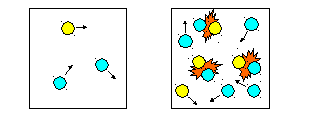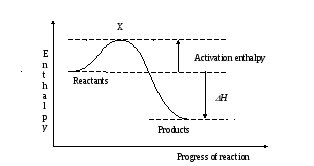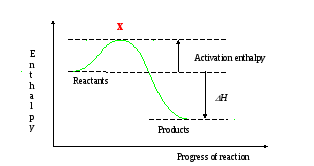# The collision theory of reactions

 Page 1/3 Date 23.04.2018 Size 244.3 Kb. #45985
1   2   3
CI 10.1 The collision theory of reactions
Reactions occur when particles of reactants collide with a certain minimum kinetic energy. (Activation enthalpy)Low concentration Higher concentration

Any factor which increases the number of collisions will increase the rate of reaction.

As particles approach and collide, K.E. is converted to P.E. and the P.E. of reactants rises.In the above exothermic reaction, existing bonds start to stretch and break and new bonds form. Only Reactants with sufficient K.E. will overcome the activation enthalpy and form products.
At higher temperatures, more of the colliding particles have enough energy to react.
Enthalpy profilesThe curved line is the energy pathway for a pair of colliding molecules (called the energy or enthalpy profile for the reaction).

X corresponds to the arrangement of atoms where old bonds are stretched and new bonds are starting to form.

Eg. For chlorine atoms and ozone, the oxygen – oxygen bonds in ozone break and a new bond between oxygen and chlorine is forming.

Cl + O3  Cl------O------O O  ClO + O

At X

(this is a very unstable arrangement and only lasts for a very short time).

The enthalpy profile is shown as a single curve (this is only true for single-step reactions). Other reactions have several steps, so the enthalpy profile would show several steps, too.
 do problems for 10.1 ‘Rates of Reactions’ p. 222-223 questions 1 to 4.

Questions

1. For each of the following reactions, say how, if at all, you would expect its rate of reaction to be affected by the following factors:

a) Temperature

b) Total pressure of gas.

c) Concentration of solution

d) Surface area of solid

 a b C A Rate will increase with temperature Rate will increase with temperature Rate will increase with temperature B Rate of forward reaction not affected Rate increases Rate of forward reaction not affected C Increasing the concentration of acid will increase the rate Solutions not involved Increasing the concentration of peroxide will increase the rate D The more finely divided the magnesium, the faster the rate The more finely divided the catalyst the faster the rate Solids not involved

2.) Both the acid and the enzyme can act as catalysts for the hydrolysis of a protein.

1. a) The greater the concentration of reactants, the greater the rate of collisions and hence the faster the reaction proceeds.

3 b) A change of temperature has little effect. Most collisions result in a reaction.

4 a) B and C b) A and D c) D d) B

e) B f) D

CI 10.2: The effect of temperature on rate
For many reactions, increasing the temperature by 10oC will DOUBLE the rate of reaction.
Extending the collision theory of reactions

Consider the reaction in the Haber process for making ammonia:

N2 (g) + 3H­2 (g) 2NH3 (g)
The collision theory says that N­2 and H2 will only react when they collide. The more frequently they collide, the faster the rate of reaction. Increasing the pressure brings H2 and N2 closer together, so they collide more often. Increasing the temperature makes molecules move faster, increasing the frequency of collisions.
How much more frequently do molecules collide, when temperatures rise?

The average speed of molecules is proportional to the square root of the absolute temperature.

Example: Temperature rise: 300 K to 310 K

Increase in average speed of molecules: (310/300)1/2

= 1.016

(this is 101.6%...so an increase of 1.6% faster)

Particles must also react with a certain minimum kinetic energy.

In the Haber process, (reaction between H2 and N2)

at 300 K only 1 in 1011 collisions between H2 and N2 results in a reaction!

Even at 800 K only 1 in 104 collisions results in a reaction.

The collision theory says:

Reactions occur when molecules collide with a certain minimum kinetic energy. The more frequent these collisions, the faster the rate of reaction.

The energy needed to overcome the energy barrier is called the activation enthalpy, for the reaction. It is the energy needed to start breaking bonds in the colliding molecules, so that collisions can lead to reactions.